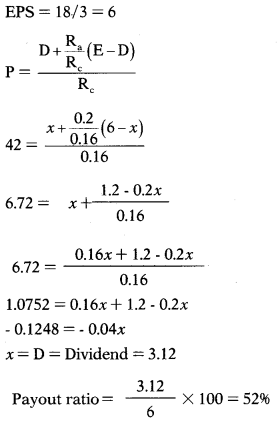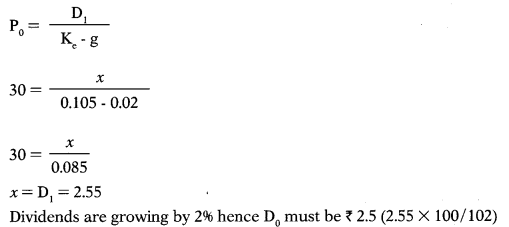# Dividend Policy – Financial Management MCQ

Dividend Policy – CS Executive Financial and Strategic Management MCQ Questions with Answers you can quickly revise the concepts.

## Dividend Policy – Financial Management MCQ

Question 1.
Dividend policy determines –
(A) what portion of earnings will be paid out to stock holders
(B) what portion will be retained in the business to finance long-term growth.
(C) Only (A) not (B)
(D) Both (A) and (B)
(D) Both (A) and (B)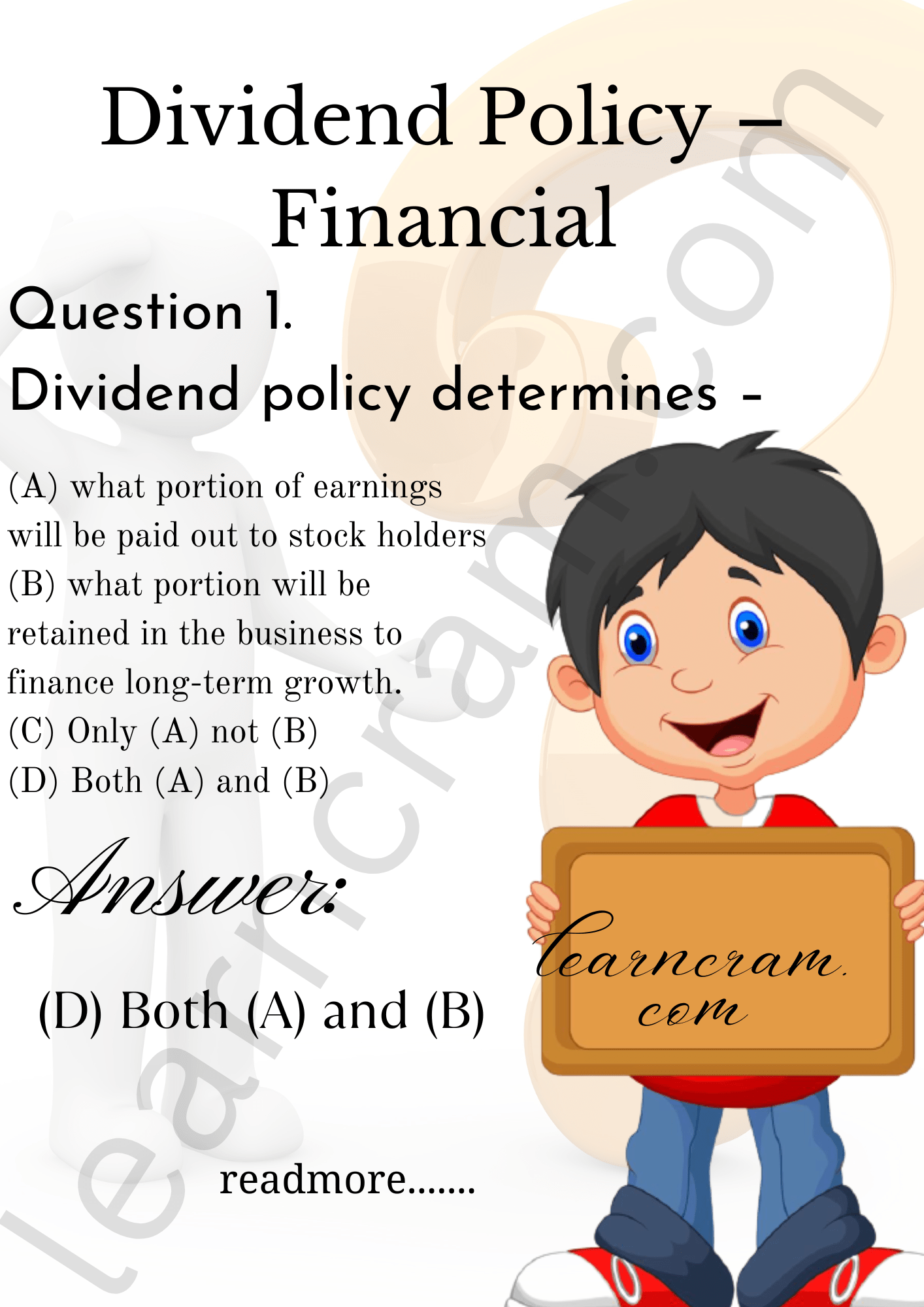Question 2.
Dividend constitutes the cash flow that accrues to -…………..
(A) Holders
(B) Equity holders
(C) Bond holders
(D) All of the above
(B) Equity holders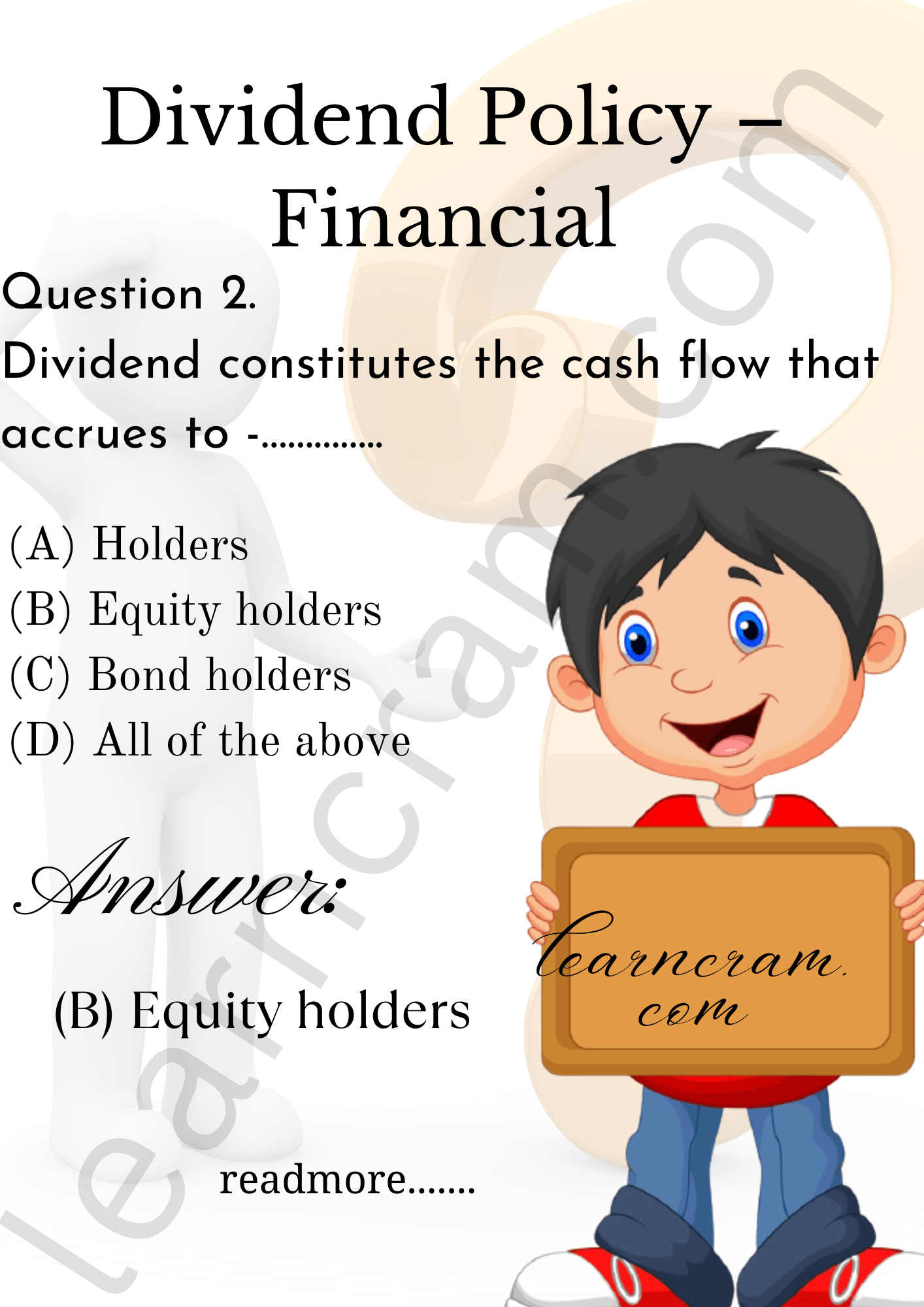Question 3.
Which of the following factor will affect the dividend policy of the firm?
1. Insufficiency of cash
2. Firms contractual obligation
3. Ratio of debt to equity
Select the correct answer from the options given below.
(A) 1 and 3 only
(B) 2 and 4 only
(C) 2, 3 and 4
(D) 1,2, 3 and 4
(D) 1,2, 3 and 4

Question 4.
Retained earnings are –
(A) An indication of a company’s liquidity.
(B) The same as cash in the bank.
(C) Not important when determining dividends.
(D) The cumulative earnings of the company after dividends.
(D) The cumulative earnings of the company after dividends.

Question 5.
In retention growth model, percent of net income firms usually pay out as shareholders dividends, is classified as –
(A) Payout ratio
(B) Payback ratio
(C) Growth retention ratio
(D) Present value of ratio
(A) Payout ratio

Question 6.
Which of the following is an argument for the relevance of dividends?
(A) Informational content.
(B) Reduction of uncertainty.
(C) Some investor’s preference for current income.
(D) All of the above.
(D) All of the above.

Question 7.
As per Modigliani-Miller hypothesis of dividend irrelevance price of share at year zero is –
(A) D0 + P0/1 + Ke
(B) (D1 + P1)× (1 + Ke)
(C) D1 + P/1+Ke
(D) 1-(D0 + P0)÷Ke
(C) D1 + P/1+Ke

Question 8.
All of the following are true of stock splits except:
(A) Market price per share is reduced after the split.
(B) The number of outstanding shares is increased.
(C) Retained earnings are changed.
(D) Proportional ownership is unchanged.
(C) Retained earnings are changed.

Question 9.
Which of the following techniques does not reward shareholders for investing in a company?
(B) Offering non-pecuniary benefits
(C) Making a rights issue
(D) Offering a scrip dividend
(C) Making a rights issue

Question 10.
Forecast by analysts, retention growth model and historical growth rates are methods used for an –
(A) Estimate future growth
(B) Estimate option future value
(C) Estimate growth ratio
(D) Estimate option present value
(A) Estimate future growth

Question 11.
The repurchase of stock is considered …………. decision rather than ……….. decision.
(A) an investment; a financing
(B) financing; an investment
(C) an investment; a dividend
(D) a dividend; a financing
(B) financing; an investment

Question 12.
If OML Corporation buyback ten percent of its outstanding common stock from the secondary market, the result would be –
(A) A decline in EPS.
(B) An increase in cash.
(C) A decrease in total assets.
(D) An increase in the number of stock-holders.
(C) A decrease in total assets.

Question 13.
Historical growth rates, analysis fore-casts and retention growth model are approaches to estimate:
(A) Net present value of gain
(B) Growth rate
(C) Growth gain
(D) Discounted gain
(B) Growth rate

Question 14.
The primary goal of a publicly-owned firm interested in serving its stockholders should be to
(A) Maximize expected total corporate profit
(B) Maximize expected EPS
(C) Maximize the stock price per share
(D) Maximize expected net income.
(C) Maximize the stock price per share

Question 15.
A decrease in a firm’s willingness to pay dividends is likely to result from an increase in its –
(A) Earnings stability
(C) Profitable investment opportunities
(D) Collection of accounts receivable.
(C) Profitable investment opportunities

Question 16.
Payout ratio is subtracted from one to calculate –
(A) Growth ratio
(B) Present value ratio
(C) Retention ratio
(D) Future value ratio
(C) Retention ratio

Question 17.
Which of the following would not have an influence on the optimal dividend policy?
(A) The possibility of accelerating or delaying investment projects.
(B) A strong share holders’ preference for current income versus capital gains.
(C) The costs associated with selling new common stock.
(D) All of the statements above can have an effect on dividend policy.
(D) All of the statements above can have an effect on dividend policy.

Question 18.
A stock split will cause a change in the toted amounts shown in which of the following balance sheet accounts?
(A) Cash
(B) Common stock
(C) Paid-in capital
(D) None of the above
(D) None of the above

Question 19.
You currently own 100 shares of stock in Baba Ltd. The stock currently trades at ₹ 120 a share. The company is contemplating a 2:1 stock split. Which of the following best describes your position after the proposed stock split takes place?
(A) You will have 200 shares of stock, and the stock will trade at or near ₹120 a share.
(B) You will have 200 shares of stock, and the stock will trade at or near ₹60 a share.
(C) You will have 100 shares of stock, and the stock will trade at or near ₹60 a share.
(D) You will have 50 shares of stock, and the stock will trade at or near ₹60 a share.
(B) You will have 200 shares of stock, and the stock will trade at or near ₹60 a share

Question 20.
Consider following two statements:
(1) Buyback can be used by companies to defend against hostile takeovers since they increase the proportion of debt in a firm’s capital structure.
(2) After a 3-for-1 stock split, a company’s price per share will fall and its number of shares outstanding will rise total value remaining the same.
Which of the above statement is correct? “%
(A) (2) only
(B) Neither (1) nor (2)
(C) (1) only
(D) Both (1) and (2)
(D) Both (1) and (2)

Question 21.
The dividend growth model can be used to compute the cost of equity for a firm in which of the following situations?
I. Firms that have a 100% retention ratio.
II. Firms that pay a constant dividend.
III. Firms that pay an increasing dividend
IV. Firms that pay a decreasing dividend.
Select correct answer from the options given below.
(A) I & II only
(B) II & III only
(C) II,III & IV only
(D) I, II & III only
(C) II,III & IV only

Question 22.
If markets are in equilibrium, which of the following will occur:
(A) Each investment’s expected return should equal its realized return
(B) Each investment’s expected return should equal its required return.
(C) Each investment should have the same realized return.
(D) All of the statements above are correct
(B) Each investment’s expected return should equal its required return.

Question 23.
Regular Dividend Policy means -……….
(A) investors get dividend at usual rate.
(B) reserve fund is created to pay fixed amount of dividend
(C) payment of low dividend per share constantly plus extra dividend in the year when the company earns high profit.
(D) All of the above
(A) investors get dividend at usual rate.

Question 24.
Which of the following examples best represents a passive dividend policy?
(A) The firm sets a policy such that the proportion of dividends paid from net income remains constant.
(B) The firm pays dividends with what remains of net income after taking acceptable investment projects.
(C) The firm sets a policy such that the quantity (dollar amount per share) of dividends paid from net income remains constant.
(D) All of the above are examples of various types of passive dividend policies
(B) The firm pays dividends with what remains of net income after taking acceptable investment projects.

Question 25.
Modigliani and Miller argue that the dividend decision
(A) is irrelevant as the value of the firm is based on the earning power of its assets.
(B) is relevant as the value of the firm is not based just on the earning power of its assets.
(C) is irrelevant as dividends represent cash leaving the firm to shareholders, who own the firm anyway.
(D) is relevant as cash outflow always influences other firm decisions
(A) is irrelevant as the value of the firm is based on the earning power of its assets.

Question 26.
Constant payout ratio means –
(A) Declaration same bonus ratio every year.
(B) The payment of fixed percentage of earning as dividend every year.
(C) Constantly paying same dividend if EPS is same for all the year.
(D) None of the above
(B) The payment of fixed percentage of earning as dividend every year.

Question 27.
Dividend policy is determined by the –
(A) Shareholders in AGM
(B) CEO of the company
(C) Board of directors
(D) Ministry of Corporate Affairs
(C) Board of directors

Question 28.
How you calculate Dividend Cover Ratio?
(A) PAT ÷ Dividend
(B) Dividend ÷ PAT
(C) EBIT ÷ Dividend
(D) Dividend ÷ EBIT
(A) PAT ÷ Dividend

Question 29.
Investors may be willing to pay a premium for stable dividends because of the informational content of ………. the desire of investors for………. and certain
(A) institutional considerations; dividends; current income.
(B) dividends; current income; institutional considerations.
(C) current income; dividends; institutional considerations.
(D) institutional considerations; current income; dividends
(B) dividends; current income; institutional considerations.

Question 30.
EPS ratio measures –
(A) Earning of the company to ratio of debt and equity
(B) Social earning per individual
(C) The profit available for the equity shareholders on a per share basis.
(D) Profit earned by the enterprises by employment various assets.
(C) The profit available for the equity shareholders on a per share basis.

Question 31.
A ………….. is a payment of additional shares to shareholders in lieu of cash.
(A) Stock split
(B) Stock dividend
(C) Extra dividend
(D) Regular dividend
(B) Stock dividend

Question 32.
Which of the following is correct formula to calculate P/E Ratio?
(A) Market Price ÷ EPS
(B) EPS ÷ Market Price
(C) PAT ÷ EPS
(D) EPS ÷ PAT
(A) Market Price ÷ EPS

Question 33.
A …………… occurs when there is an increase in the number of shares out-standing by reducing the par value of stock.
(A) Stock split
(B) Stock dividend
(C) Extra dividend
(D) Regular dividend
(A) Stock split

Question 34.
The P/E ratio reflects –
(A) the market’s confidence in the company’s management effectiveness.
(B) Effectiveness of capital budget process.
(C) the market’s confidence in the company’s stakeholders.
(D) the market’s confidence in the company’s equity.
(D) the market’s confidence in the company’s equity.

Question 35.
A ……………. is the expected cash dividend that is normally paid to shareholders.
(A) Stock split
(B) Stock dividend
(C) Extra dividend
(D) Regular dividend
(D) Regular dividend

Question 36.
Myron Gordon believe that the required return on equity increases as the dividend payout ratio is decreased. Their argument is based on the assumption that
(A) Investors are indifferent between dividends and capital gains.
(B) Investors require that the dividend yield and capital gains yield equal a constant.
(C) Capital gains are taxed at a higher rate than dividends.
(D) Investors view dividends as being less risky than potential future capital gains.
(D) Investors view dividends as being less risky than potential future capital gains.

Question 37.
………….. is a non-recurring dividend paid to shareholders in addition to the regular dividend.
(A) A stock split
(B) A stock dividend
(C) An extra dividend
(D) A regular dividend
(C) An extra dividend

Question 38.
In the real world, we find that dividends –
(A) Usually exhibit greater stability than earnings.
(B) Fluctuate more widely than earnings
(C) Tend to be a lower percentage of earnings for mature firms
(D) Are usually set as a fixed percentage of earnings
(A) Usually exhibit greater stability than earnings.

Question 39.
What method of stack repurchase occurs when the buyer purchases securities through a brokerage house?
(A) Dutch-auction
(B) Fixed-price
(C) Open-market
(D) Fair-warning
(C) Open-market

Question 40.
What method of stock repurchase occurs when the buyer seeks bids within a specified price range and accepts the lowest price that will allow it to acquire the entire block of securities desired?
(A) Dutch-auction
(B) Fixed-price
(C) Open-market
(D) Fair-warning
(A) Dutch-auction

Question 41.
Which of the following is correct formula to calculate dividend payout ratio?
(A) DPS ÷ EPS
(B) EPS ÷ DPS
(C) Market Price ÷ EPS
(D) EPS ÷ Market Price
(A) DPS ÷ EPS

Question 42.
The shareholders of your firm anticipate receiving a regular dividend that is consistent with past dividend policies. What benefit occurs to shareholders if the firm repurchases shares with the same total quantity of money that would have been spent on dividends? Assume that the P/E ratio is maintained with either scenario.
(A) Shareholders can postpone or reduce taxes (assuming a lower capital gain rate).
(B) It is cheaper for shareholders to sell existing shares for cash than it costs to reinvest cash dividends into existing shares.
(C) The current shareholders benefit because there are a greater number share holders than if the firm pays a cash dividend.
(D) There is no benefit as shareholders will not be receiving any cash
(A) Shareholders can postpone or reduce taxes (assuming a lower capital gain rate).

Question 43.
Dividend payout ratio – ……………
(A) expresses the relationship between what is available as earnings per share and what is actually paid in the form of dividends out of available earnings.
(B) is a good measure of the dividend policy of the company.
(C) Both (A) and (B)
(D) Neither (A) nor (B)
(C) Both (A) and (B)

Question 44.
A dividend reinvestment plan (DRIP) is –
(A) An optional plan, provided by brokerage firms, allowing unit holders to automatically reinvest dividend payments in additional units of the mutual fund.
(B) An optional plan, provided by mutual funds, allowing unit holders to automatically reinvest dividend payments in additional units of the mutual funds.
(C) A mandatory plan, provided by brokerage firms, where shareholders are automatically reinvesting dividend payments in additional units of the mutual fund at a reduced price.
(D) A mandatory plan, provided by mutual funds, where unit holders are automatically reinvesting dividend payments in additional emits of the mutual funds at a reduced price.
(B) An optional plan, provided by mutual funds, allowing unit holders to automatically reinvest dividend payments in additional units of the mutual funds.

Question 45.
Which of the following is correct formula to calculate dividend yield ratio?
(A) DPS Question Market Price × 100
(B) (1 – EPS) Question Market Price
(C) EPS Question Market Price × 100
(D) (Market Price × 100) ÷ DPS
(A) DPS Question Market Price × 100

Question 46.
Which of the following is not a reason that DeStore.com would prefer to pay a stock dividend rather than a regular cash dividend?
(A) It decreases the supply of shares and enhances shareholder wealth.
(B) It may conserve cash for other firm needs.
(C) It will reduce the stock price into what management perceives as a more beneficial trading range.
(D) It may convey information about the firm to investors that it cannot convey credibly otherwise.
(A) It decreases the supply of shares and enhances shareholder wealth.

Question 47.
Some ratios are given below:
I. EPS
II. P/E Ratio
III. Net Profit Ratio
IV. DPS
V. Dividend Yield Ratio
Which of the above ratio can be classified as market test ratio?
(A) Except V all other
(B) II & V only
(C) I, III & IV
(D) All except III
(D) All except III

Question 48.
The ……….. is the proportion of earnings that are paid to common shareholders in the form of a cash dividend.
(A) Retention rate
(B) 1 + Retention rate
(C) Growth rate
(D) Dividend payout ratio
(D) Dividend payout ratio

Question 49.
All of the following statements are
true regarding ratios that analyze a stock investment except
(A) In general, an increased P/E ratio indicates increased investor confidence in the future of the company.
(B) Shareholders who invest primarily to receive dividends pay special attention to the dividend yield ratio.
(C) Many experts argue that book value is the most useful ratio for investment analysis.
(D) Two ways for shareholders to earn a return on a share investment are receiving dividends and selling the stock investment at again.
(C) Many experts argue that book value is the most useful ratio for investment analysis.

Question 50.
The dividend payout ratio describes:
(A) The proportion of earnings paid as dividends
(B) The relationship of dividends per share to market price per share
(C) The percentage change in dividends this year compared to last year
(D) Dividends as a percentage of the price/earnings ratio
(A) The proportion of earnings paid as dividends

Question 51.
Which of the following factors is most likely to explain why a company decides to increase its annual dividend?
(A) A firm belief by management that dividends represent a residual payment
(B) A large number of desirable projects.
(C) A large proportion of its shares are owned by institutional investors
(D) Pecking order theory
(C) A large proportion of its shares are owned by institutional investors

Question 52.
Company J and Company K each recently reported the same EPS. Company J’s stock, however, trades at a higher price. Which of the following statements is most- correct?
(A) Company J must have a higher P/E ratio.
(B) Company J must have a higher market to book ratio.
(C) Company J must be riskier.
(D) All of the statements above are correct.
(A) Company J must have a higher P/E ratio.

Question 53.
Which of the following statements lends most support to the theory that dividend payments are irrelevant to the value of ordinary shares?
(A) Shareholders making homemade dividends face dealing costs
(B) Shareholders are concerned with total earnings rather than with the split between distributed and retained earnings.
(C) Investors’ discount rates increase with time due to uncertainty.
(D) Firms have particular clienteles due to their dividend policy
(C) Investors’ discount rates increase with time due to uncertainty.

Question 54.
Which of the following is market test ratio?
(A) Basic Defence Interval
(B) Debt Service Coverage
(C) Dividend Yield Ratio
(D) All of the above
(C) Dividend Yield Ratio

Question 55.
Which of the following statements is consistent with dividend irrelevance theory?
(A) Investment decisions are the sole determinant of shareholder wealth
(B) Making homemade dividends causes investors to incur transaction costs
(C) Companies with stable dividend policies build up shareholder clienteles
(D) Investors like to maintain the real value of their dividend payments.
(A) Investment decisions are the sole determinant of shareholder wealth

Question 56.
While calculating dividend cover for preference shares numerator should be taken as
(A) EBIT
(B) Profit available for equity shareholder
(C) PAT
(D) PAT + Depreciation
(C) PAT

Question 57.
Which of the following statements about the dividend growth model are true?
1. The model prices shares on the basis of the present value of expected future dividends.
2. The model relies on the ability to predict a constant future growth rate for dividend payments.
3. The dividend growth model can accommodate future changes in shareholder’s required rate of return
Select the correct answer from the options given below.
(A) Only 1 and 2 are correct
(B) Only 2 and 3 are correct
(C) 1, 2 and 3 are correct
(D) Only 3 is correct
(A) Only 1 and 2 are correct

Question 58.
………… reflects the market’s confidence in the company’s equity.
(A) P/E ratio
(B) Net profit ratio
(C) Cash profit ratio
(D) Total assets turnover ratio
(A) P/E ratio

Question 59.
Financial signalling has been raised as an argument in the battle over the relevancy of dividends. Which of the following statements concerning dividends is most likely to be voiced by someone using the financial signalling argument?
(A) A dividend decrease should be viewed by investors as “goodnews.” The dividend decrease acts to add conviction to the statement that the firm has better uses for the earnings of the company than the stockholders.
(B) Reported accounting earnings of a company, not dividends, are a proper reflection or signal of the company’s economic earnings.
(C) The price of a firm’s stock should react unfavourably to an increase in dividends.
(D) Cash dividends speak louder than words when it comes to conveying information about management’s expectations of the future
(D) Cash dividends speak louder than words when it comes to conveying information about management’s expectations of the future

Question 60.
…. is a good measure of the dividend policy of the company.
(A) Dividend Payout Ratio
(B) Price Earnings Ratio
(C) Earnings Per Share
(D) None of the above
(A) Dividend Payout Ratio

Question 61.
Which of the following statement is correct?
(A) A company may, if so authorized by its articles, pay dividends in proportion to the amount paid-up on each share.
(B) Dividend cannot be paid on calls-in-advance.
(C) All the provisions of the Companies Act, 2013 that are applicable to final dividend are also applicable to interim dividend.
(D) All of the above
(D) All of the above

Question 62.
As per provisions of the Companies Act, 2013, dividend can be paid –
1. Out of current profit
2. Out of revaluation reserve
3. Out of profits of previous financial years
4. Out of money provided by the Central or State Government
5. Out of free reserve
Select the correct answer from the options given below.
(A) 1 and 5 only
(B) 1,2, 3 & 5
(C) 1, 3 and 5 only
(D) 1,3, 4 and 5
(D) 1,3, 4 and 5

Question 63.
As per Section 128 of the Companies Act, 2013, a company may, before the declaration of any dividend in any financial year, transfer to the reserves of the company.
(A) 25% of its profit
(B) 10% of its profit before tax
(C) such percentage of its profits for that financial year as it may consider appropriate
(D) such percentage of its profits for that financial year as equity shareholder may consider appropriate
(C) such percentage of its profits for that financial year as it may consider appropriate

Question 64.
As per Rule 7 of the Companies (Declaration & Payment of Dividend) Rules, 2014, in the event of inadequacy or absence of profits in any year, a company may declare dividend out of surplus subject to the fulfilment of the condition that rate of dividend declared shall not exceed the average of the rates at which dividend was declared by it in the immediately preceding that year.
(A) 5 years
(B) 10 years
(C) 3 years
(D) 4 years
(C) 3 years

Question 65.
As per Rule 7 of the Companies (Declaration & Payment of Dividend) Rules, 2014, in the event of inadequacy or absence of profits in any year, a company may declare dividend out of surplus subject to the fulfilment of the condition that total amount to be drawn from such accumulated profits shall not exceed as appearing in the latest audited financial statement.
(A) 1/10th of the total assets
(B) 1 /5th of the sum of its paid-up share capital
(C) 1/10th of the sum of its paid-up share capital and free reserves
(D) 1 / 5th of the sum of its paid-up share capital and free reserves
(C) 1/10th of the sum of its paid-up share capital and free reserves

Question  66.
As per Rule 7 of the Companies (Declaration & Payment of Dividend) Rules, 2014, in the event of inadequacy or absence of profits in any year, a company may declare dividend out of surplus subject to the fulfilment of the condition the balance of reserves after such withdrawal shall not
fall below as appearing in the latest audited financial statement.
(A) 10% of its paid-up share capital
(B) 15% of its paid-up share capital
(C) 15% of its paid-up share capital and free reserve
(D) 10% of its paid-up share capital and free reserve
(C) 15% of its paid-up share capital and free reserve

Question 67.
As per the provisions of the Companies Act, 2013, the amount of the dividend, including interim dividend, shall be deposited in a scheduled bank in a separate account within ………….. from the date of declaration of such dividend.
(A) 30 days
(B) 15 days
(C) 10 days
(D) 5 days
(D) 5 days

Question 68.
After declaration of dividend, the company has to pay dividend within of declaration of dividend. If amount of dividend remains unpaid or unclaimed for 30 days of declaration of dividend, then in next ……….. the company has to transfer the amount unclaimed to the to a special account in any scheduled bank to be called the “Unpaid Dividend Account”.
(A) 5 days; 5 days
(B) 30 days; 7 days
(C) 30 days; 5 days
(D) 10 days; 7 days
(B) 30 days; 7 days

Question 69.
The shareholders may desire that their dividends be credited directly to their bank account. The request will be made in a form duly filled and sent to the company. This is known as –
(A) Dividend Cheque
(B) Dividend Request Form
(C) Dividend Mandate
(D) Pay Dividend Form
(C) Dividend Mandate

Question 70.
According to ………….. dividend policy is strictly a financing decision.
(A) Ezra Solomon
(B) Modigliani-Miller
(C) Marshall
(D) John Miller
(A) Ezra Solomon

Question 71.
Professor James E. Walter has developed a theoretical model which shows the relationship between
(A) Dividend policies and common stock prices.
(B) Earning policies and common stock prices.
(G) Cost of capital and common stock prices.
(D) Earning policies and dividend policies
(A) Dividend policies and common stock prices.

Question 72.
As per Walter’s Model in case of growth firm –
(A) Dividend policies are irrelevant
(B) Optimal payout ratio is 100%
(C) Payout ratio is irrelevant
(D) Optimal payout ratio is nil
(D) Optimal payout ratio is nil

Question 73.
……………. suggests that the market price of share is the present value of future dividends.
(A) Walter’s model
(B) MM Model
(C) Grodon’s model
(D) General knowledge
(C) Grodon’s model

Question 74.
As per Grodon’s Model payout ratio is irrelevant in case of –
(A) Growth firm
(B) Normal firm
(C) Declining firm
(D) All of the above
(B) Normal firm

Question 75.
How market price will be calculated by using the dividend growth model?
(A) P0 = D1÷(Ke – g)
(B) P0 = (Ke – g) ÷ D1
(C) P0 = (1+D1)÷(Ke-g)
(D) P0 = (1 + D1) × (Ke – g)
(A) P0 = D1÷(Ke – g)

Question 76.
Consider following two statements:
(I) A company with large portion of inside ownership, all of whom are high-income individuals.
(II) A growth company with an abundance of good investment opportunities.
For each of the company described above, would you expect it to have a high or low dividend payout ratio?
(A) low dividend payout ratio for both companies
(B) high dividend payout ratio for both companies
(C) low dividend payout ratio for company mentioned in Statement (I) and high dividend payout ratio for company mentioned in Statement (II)
(D) high dividend payout ratio for company mentioned in Statement (I) and low dividend payout ratio for company mentioned in Statement (II)
(A) low dividend payout ratio for both companies

Question 77.
Consider following two statements:
(I) A company is experiencing ordinary growth and has high liquidity and much unused borrowing capacity.
(II) A company with volatile and high business risk.
For each of the company described above, would you expect it to have a high or low dividend payout ratio?
(A) low dividend payout ratio for both companies
(B) high dividend payout ratio for both companies
(C) low dividend payout ratio for company mentioned in Statement (I) and high dividend payout ratio for company mentioned in Statement (II)
(D) high dividend payout ratio for company mentioned in Statement (I) and low dividend payout ratio for company mentioned in Statement (II)
(D) high dividend payout ratio for company mentioned in Statement (I) and low dividend payout ratio for company mentioned in Statement (II)

Question 78.
If you are calculating market price by using Gordon’s Model, increasing payout ratio other things renaming the same will –
(A) Increase the price per share
(B) Decrease the price per share
(C) Will not have any effect on price of the share
(D) Price will remain constant.
(C) Will not have any effect on price of the share

Question 79.
As per Gordon’s Model whether com­pany adopts 50%, 80% or any other payout ratio, market price will remain same when -…………..
(A) K>r
(B) Ke<r
(C) K=r
(D) K>Rf
(C) K=r

Question 80.
If you are calculating market price by using dividend growth method D1 ÷ (Ke – g) increase in growth rate leads to –
(A) Fall in market price of stock
(B) Increase in market price of stock
(C) No change in market price of stock
(D) None of the above
(B) Increase in market price of stock

Question 81.
Company A and Company B both cal­culates their market price by using Walter’s formula. Both companies will have same market price if –
(A) Ra > Rc and retention ratio of Com­pany A is more than retention ratio of Company B.
(B) Ra < Rc and retention ratio of Com­pany B is more than retention ratio of Company A.
(C) Ra = Rc whether retention ratio is same or different for both the com­panies.
(D) All of the above
(C) Ra = Rc whether retention ratio is same or different for both the com­panies.

Question 82.
As per Walter’s Model when Ra < Rc in­crease in dividend payout ratio will lead to –
(A) Increase in market price
(B) Decrease in market price
(C) No change in market price
(D) None of the above
(A) Increase in market price

Question 83.
As per Walter’s Model when Ra < Rc decrease in retention ratio will lead to –
(A) Increase in market price
(B) Decrease in market price
(C) No change in market price
(D) None of the above
(A) Increase in market price

Question 84.
As per Walter’s Model when R = R market price will remain same when –
(A) Retention ratio increases
(B) Retention ratio decreases
(C) Retention ratio increase or decreases
(D) None of the above
(C) Retention ratio increase or decreases

Question 85.
As per Walter’s Model when Ra > Rc in­crease in dividend payout ratio will lead to –
(A) Increase in market price
(B) Decrease in market price
(C) No change in market price
(D) None of the above
(B) Decrease in market price

Question 86.
As per Walter’s Model when Ra > Rc decrease in retention ratio will lead to –
(A) Increase in market price
(B) Decrease in market price
(C) No change in market price
(D) No change in market price
(B) Decrease in market price

Question 87.
Which of the following is correct formula to calculate market price as per MM Model?
(A) P0 = (1 + Ke) ÷ (D1 + P1)
(B) P0 = (D1 + P1) × (1 + Ke)
(C) P0 = (D1+ P1) ÷ (1 + Ke)
(D) P0 = (D1 + P1) ÷ (1 – Ke)
(C) P0 = (D1+ P1) ÷ (1 + Ke)

Question 88.
As per MM Model total value of firm remains same whether it declares dividend or not. You are required to state if dividend is declared the market price per share as per MM Model –
(A) Increase
(B) Decreases
(C) Remain constant
(D) None of the above
(A) Increase

Question 89.
Which one of the following is a non-cash payment made by a firm to its shareholders that dilute the value of each share of stock outstanding?
(A) reverse stock split
(B) cash distribution
(C) stock dividend
(D) regular dividend
(C) stock dividend

Question 90.
Market price = ?
(A) D1 ÷ (Ke – g)
(B) EPS × P/E Ratio
(C) (D1 + P1) ÷ (1 + Ke)
(D) All of the above
(D) All of the above

Question 91.
The date by which a shareholder must be recorded as the share owner in order to receive a declared dividend is called the:
(A) ex-rights date
(B) ex-dividend date
(C) date of record
(D) date of payment
(C) date of record

Question 92.
The target payout ratio is:……………
(A) a firm’s preferred rate of dividend growth.
(B) the inverse of a firm’s equity multiplier.
(C) the preferred number of dividend payments per year divided by 12.
(D) a firm’s long-term desired dividend-to-earnings ratio.
(D) a firm’s long-term desired dividend-to-earnings ratio.

Question 93.
The difference between the highest and lowest prices at which a stock has sold is called the stock’s:
(A) Average price
(D) Opening price

Question 94.
Which one of the following statements concerning cash dividends is correct?
(A) The chief financial officer of a corporation determines whether or not a dividend will be paid.
(B) A dividend is not a liability of a firm until it has been declared.
(C) If a firm has paid regular quarterly dividends in the past it is legally obligated to continue doing so.
(D) Cash dividends always reduce the paid-in capital account balance
(B) A dividend is not a liability of a firm until it has been declared.

Question 95.
The fact that flotation costs can be significant is justification for:
(A) A firm to issue larger dividends than their closest competitors.
(B) Maintaining a constant dividend policy even when profits decline significantly.
(C) Maintaining a high dividend policy.
(D) Maintaining a low dividend policy and rarely issuing extra dividends.
(D) Maintaining a low dividend policy and rarely issuing extra dividends.

Question 96.
When a firm is short of cash yet it wishes to distribute something to shareholders, it should consider –
(A) Cash dividend.
(B) Liquidating dividend
(C) Stock dividend
(D) None of the above
(C) Stock dividend

Question 97.
A company wants to buy back stock. How will this impact the company and its stock?
(A) The company makes more money because management owns more stock.
(B) Other investors make less money because management can pay more dividends to internal shareholders before external shareholders.
(C) Because there are fewer shares in the open market, the price of the shares goes up.
(D) The net income of the company will go up because of the increase in stocks
(C) Because there are fewer shares in the open market, the price of the shares goes up.

Question 98.
Which of the following would ultimately give the greatest benefit to stockholders?
(B) The issuance of a bond
(C) A stock split
(D) The issuance of new stock

Question 99.
Match the following: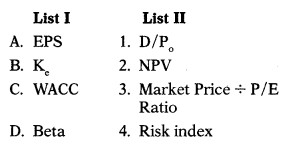Select the correct answer from the options given below.(B)

Question 100.
Required return × Retention Ratio=?
(A) Ke (Cost of equity)
(B) WACC
(C) B (Beta)
(D) g (Growth Rate)
(D) g (Growth Rate)

Question 101.
The shares of company are selling at ₹ 45 per share. The firm had paid dividend @ ₹ 4.5 per share last year. The estimated growth of the company is approximately 5% per year. Determine the estimated market price of the equity share if the anticipated growth rate of the firm rises to 8%.
(A) ₹ 60.45
(B) ₹ 64.80
(C) ₹ 65.70
(D) ₹ 80.35
(B) ₹ 64.80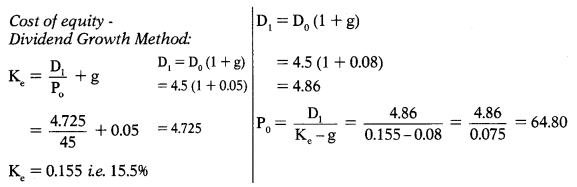Question 102.
The shares of company are selling at ₹ 90 per share. The firm had paid dividend @ ₹ 9 per share last year. The estimated growth of the company is approximately 5% per year. Determine the estimated market price of the equity share if the anticipated growth rate of the firm falls to 3%.
(A) ₹ 70.84
(B) ₹ 84.70
(C) ₹ 74.16
(D) ₹ 52.34
(C) ₹ 74.16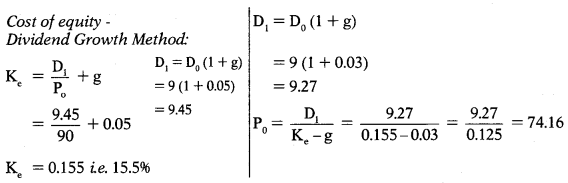Question 103.
Details regarding A Ltd. are given below:
E = ₹ 24
K = 11%
r = 12%
If retention ratio is 80%, market price as per Gordon’s Model is –
(A) ₹ 340.68
(B) ₹ 346.82
(C) ₹ 324.65
(D) ₹ 342.86
(D) ₹ 342.86Question 104.
Details regarding B Ltd. are given below:
E = ₹ 24
K = 13.20%
r = 14.40%
If retention ratio is 50%, market price as per Gordon’s Model is –
(A) ₹ 200
(B) ₹ 300
(C) ₹ 400
(D) ₹ 600
(A) ₹ 200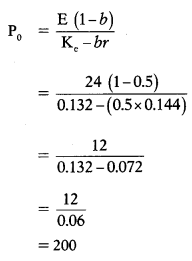Question 105.
Details regarding C Ltd. are given below:
E = ₹ 14.4
Ke =7.92%
r = 8.64%
If retention ratio is 90%, market price as per Gordon’s Model is –
(A) ₹ 200
(B) ₹ 25
(C) ₹ 100
(D) ₹ 130
(C) ₹ 100Question 106.
Following details are available for G Ltd.:
E = ₹ 22
Ke = 12.10%
r = 12.10%
Calculate the value of equity shares when retention ratio is
(a) 50%, (b) 80% , (c) 90%.
(A) ₹183.28; ₹ 183.28; ₹ 183.28
(B) ₹181.82; ₹ 181.82; ₹ 181.82
(C) ₹ 175.26; ₹ 190.26; ₹ 130.26
(D) ₹ 200.22; ₹ 180.24; ₹ 120.18
(B) ₹181.82; ₹ 181.82; ₹ 181.82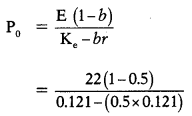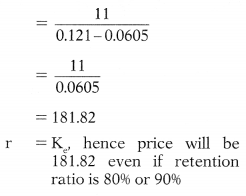Question 107.
Market price per share of WX Ltd. is ₹ 400 per share at 50% retention ratio as per Gordon’s Model. The firms cost of equity is below required rate of return. If the firm increases retention ratio –
(A) Its price will decrease and will go below ₹ 400
(B) Its price will increase and will go above ₹ 400
(C) Its price will still remain at ₹ 400
(D) None of the above
(B) Its price will increase and will go above ₹ 400Question 108.
JKL Ltd. is foreseeing a growth rate of 12% p.a. in the next 2 years. The growth rate is likely to fall to 10% for the third year and forth year. After that growth rate is expected to stabilize at 8% p.a. If .the last dividend (D0) paid was ₹ 2.7 per share and investor’s required rate of return is 16%, find out the intrinsic value per share of JKL Ltd. as of date.(A) ₹ 55.38
(B) ₹ 40.21
(C) ₹ 50.26
(D) ₹ 46.88
(B) ₹ 40.21

Question 109.
A large chemical company has been expected to grow at 14% per year for next 4 years and then to grow indefinitely at the same rate as national economy ie. 5%. The required rate of return on equity share is 12%. Assume that company paid a dividend of ₹ 2 per share last year (D0 = 2). Determine the market price of the shares today.
(A) ₹ 40.62
(B) ₹ 50.42
(C) ₹ 45.23
(D) ₹ 61.18
(A) ₹ 40.62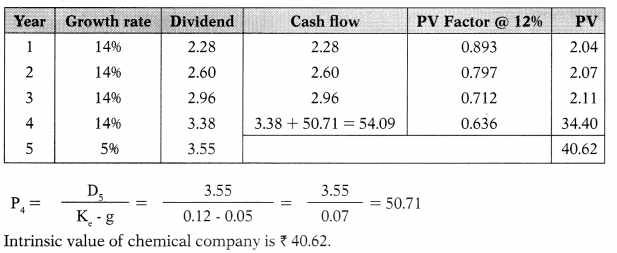Question 110.
The required rate of return of investors is 15%. ABC Ltd. declared and paid annual dividend of ₹ 4 per share. It is expected to grow @ 20% for the next 2 years and 10% thereafter. Compute the price at which the shares should sell.
PV Factors: @15% for Year 1 = 0.8696 and Year 2 = 0.7561.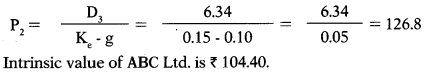Question 111.
Anurag has invested in a share whose dividend is expected to grow @15% for 5 years and there after @ 5% till life of the company. Find out the value of the share, if current dividend is ₹ 4 per share and investors required rate of return is 6%.Question 112.
The cost of capital and rate of return on investment of GOD Ltd. is 10% and 15% respectively. The company has 10 lakh shares of 10% each. Its earnings per share is ₹ 7.5. Calculate the value of the firm per share using Walter’s Model assuming all earnings are distributed as dividend.
(A) ₹ 75
(B) ₹ 100
(C) ₹ 125
(D) ₹ 150
(A) ₹ 75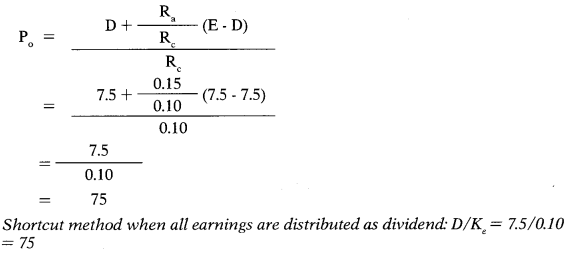Question 113.
Cost of capital of MNL Ltd. is less them rate of return on investment. Its market price per share is ₹ 62.50 as per Walter’s Model at 50% retention ratio. If firm increase its retention ratio then –
(A) Its price will fall below — ₹ 62.50
(B) Its price will increase above — ₹ 62.50
(C) Its price will increase above — ₹ 100
(D) It price will not change
(B) Its price will increase above — ₹ 62.50

Question 114.
EPS of Kalki Ltd. is ₹ 20. Its cost of capital is 16%. Internal rate of return on investment is 20% and retention ratio is 50%. What is the market price per share of Kalki Ltd. as per dividend growth model ?
(A) ₹ 164.55
(B) ₹ 176.66
(C) ₹ 166.67
(D) ₹ 167.33
(C) ₹ 166.67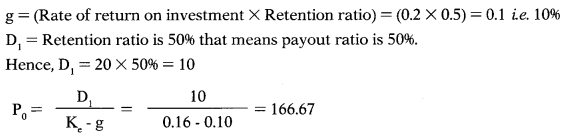Question 115.
EPS of Don Ltd. is ₹ 15. Its cost of capital is 16%. Internal rate of return on investment is 20% and retention ratio is 40%. What is the market price per share of Don Ltd. as per Walter’s Model?
(A) ₹ 103.125
(B) ₹ 105.225
(C) ₹ 108.125
(D) ₹ 110.525
(A) ₹ 103.125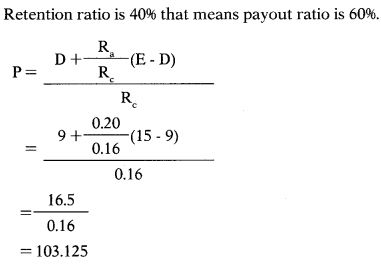Question 116.
Following details are available for PQR Ltd:
Earnings per share — ₹ 27.5
Cost of capital — 16%
Internal rate of return on investment — ₹ 20%
Retention ratio — 50%
Calculate the price per share as per Walter’s Model.
(A) ₹ 315.40
(B) ₹ 193.36
(C) ₹ 292.91
(D) ₹ 282.86
(B) ₹ 193.36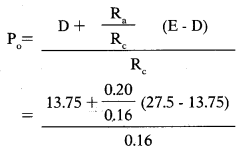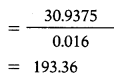Question 117.
Following details are available for PQR Ltd.:
Calculate the price per share as per Walter’s Model.
(A) ₹ 190.60
(B) ₹ 188.72
(C) ₹ 176.28
(D) ₹ 189.06
(D) ₹ 189.06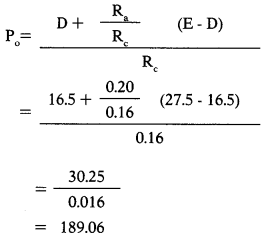Question 118.
Details regarding three companies are given below:If Walter’s Model is applied with 100% retention ratio market price per share of these companies are –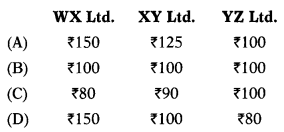(D)

Question 119.
Khemka Ltd. paid dividend per share of ₹ 3, ₹ 3.6, ₹ 4.32, ₹ 5.18 & ₹ 6.22 for last five year. What is the expected dividend for next year?
(A) ₹ 7.46
(B) ₹ 8.32
(C) ₹ 6.80
(D) ₹ 7.25
(A) ₹ 7.46

Question 120.
Details regarding three companies are given below:If Walter’s Model is applied with 50% retention ratio market price per share of these companies are(D)

Question 121.
ABC Ltd. was started a year back with a paid-up capital of ₹ 56,00,000. The other details are as under:
You are required to find out market price per share using Walter’s formula.
(A) ₹ 138.57 per share
(B) ₹ 131.25 per share
(C) ₹ 175.83 per share
(D) ₹ 185.73 per share
(B) ₹ 131.25 per shareQuestion 122.
The EPS of the Ganga Ltd. is 716. The market capitalization rate applicable to the company is 12.5%. Retained earnings can be employed to yield return of 10%. The company is considering payout ratio of 25%, 50%, 75% and 100%. Which of these, if any, would maximize the wealth of shareholders as per Walter’s Model?
(A) If payout ratio is 25%
(B) If payout ratio is 50%
(C) If payout ratio is 75%
(D) If payout ratio is zero percentage
(D) If payout ratio is zero percentage
For Ganga Ltd. Ra > Rc (12.5% > 10). It is growth firm as its rate of return on investment is greater than cost of capital. Optimal payout ratio as per Walter’s Model is nil. (in other words 100% retention policy must be followed to maximize the wealth of shareholders)

Question 123.
Prakash Ltd. has had earnings of ₹ 3.20, ₹ 3.00 & ₹ 5.50 per share for the past 3 years. Company anticipates maintaining the same dividend policy this year as the past 3 years. That dividend policy has resulted in dividends per share of ₹ 1.28, ₹ 1.20 & ₹ 2.20 for the past 3 years. It is anticipated that the next year will result in a large increase in earnings to ₹ 9.80 per share. What dividend do you expect the firm to pay in the next year?
(A) ₹ 3.92
(B) ₹ 1.56
(C) ₹ 3.12
(D) ₹ 4.68
(A) ₹ 3.92
DPS Ratio: Year 1:1.28/3.2 × 100 = 40%; Year 2:1.2/3 × 100 = 40%; Year 3:22/5.5 × 100 = 40%
Dividend for next year = 9.8 × 40% = 3.92

Question 124.
MNP Ltd. has made plans for the next year. The company will employ total assets of ₹ 25,00,000; 30% of assets being financed by debt at an interest cost of 9% p.a. The direct costs for the year are estimated at ₹ 15,00,000 and all other operating expenses are estimated at ₹ 2,40,000. Sales revenue are estimated at ₹ 22,50,000. Tax rate is assumed to be 40%.
Return on equity = ?
(A) 12.46%
(B) 15.17%
(C) 11.86%
(D) 15.98%
(B) 15.17%
Total assets = 25,00,000;
Equity = 25,00,000 × 70% = 17,50,000;
Debt = 25,00,000 × 30% = 7,50,000
22,50,000 – 15,00,000 – 2,40,000 – 67,500 = 4,42,500
PAT = 4,42,500 – 1,77,000 = 2,65,500
Return on equity = 2,65,500/17,50,000 × 100 = 15.17%

Question 125.
Joshi Corporation is growing at a constant rate of 6% per year. It has both common stock and non-participating preferred stock outstanding. The cost of preferred stock (K) is 8%. The par value of the preferred stock is ₹ 120, and the stock has a stated dividend of 10% of par. What is the market value of the preferred stock
(A) ₹ 125
(B) ₹ 120
(C) ₹ 175
(D) ₹ 150
(D) ₹ 150
12/0.08 = 150

Question 126.
A share of common stock has just paid a dividend of ₹ 2.00. If the expected long-run growth rate for this stock is 15 percent, and if investors require a 19 percent rate of return, what is the price of the stock?
(A) ₹ 57.50
(B) ₹ 62.25
(C) ₹ 71.86
(D) ₹ 64.00
(A) ₹ 57.50
D1 =2×1.15 = 2.3
2.3/(0.19 – 0.15) = 57.5

Question 127.
CPC Company’s stock is currently selling for ₹ 40 a share. The stock is expected to pay a ₹ 2 dividend at the end of the year. The stock’s dividend is expected to grow at a constant rate of 7 percent a year forever. The risk-free rate (RF) is 6 percent and the market risk premium (RM – RF) is also 6 percent. What is the stock’s beta?
(A) 1.06
(B) 1.00
(C) 2.00
(D) 0.83
(B) 1.00
Ke = 2/40 + 0.07 = 0.12 12%
K =Rf + β(Rm-Rf)
12 = 6 + 6x
6 = 6x
x = β = 1

Question 128.
Retention ratio is 0.60 and return on equity is 15.5% then growth retention model would be –
(A) 14.9%
(B) 25.84%
(C) 16.1%
(D) 9.3%
(D) 9.3%
15.5% × 0.60 = 9.3%

Question 129.
Ali Motors recently completed a 3 for 1 stock split. Prior to the split, the company had 10 Million shares outstanding and its stock price was ₹150 per share. After the split, the total market value of the company’s stock equalled 71.5 Billion. What was the price of the company’s stock following the stock split?
(A) ₹ 15
(B) ₹ 45
(C) ₹ 50
(D) ₹ 150
(C) ₹ 50
150 × 1/3 = 50

Question 130.
If payout ratio is 0.45 then retention ratio will be:
(A) 55%
(B) 1.45
(C) 0.055
(D) 100%
(A) 55%

Question 131.
Retention ratio is 0.55 and return on equity is 12.5% then growth retention model would be –
(A) 0.1195
(B) 0.06875
(C) 0.1305
(D) 0.2272
(B) 0.06875

Question 132.
Company Q is all equity financed. For each ₹ 1 of earnings, it consistently pays 30 paisa in dividends and retains 70 paisa for reinvestment. It expects to earn a rate of return of 14% on capital employed. According to the Gordon Growth Model, what would the rate of earnings growth be in future Ignore tax.
(A) 4-2%
(B) 7%
(C) 9-8%
(D) 14%
(C) 9-8%
br= g = 0.70 × 14 = 9.8%

Question 133.
DHC Ltd. is looking to purchase WIC Ltd., which has the following information: Revenue ₹ 40,00,000; EBITD ₹ 9,00,000; Basic EPS ₹ 1.40; Net assets ₹ 50,00,000 Dividends paid ₹ 0.50. Research has shown that the price-earnings ratio for companies like WIC Ltd. is 9.5. Based on that ratio, what is the value of WIC Ltd.?
(A) 23,75,000
(B) 85,50,000
(C) 50,00,000
(D) 66,50,000
(D) 66,50,000
Market Price = P/E Ratio × EPS = 9.5 × 1.4 = 13.3;
50,00,000×13.3/10 = 66,50,000

Question 134.
Market price of Jhakas Ltd. is ₹200 per share as per Gordon Model. EPS is ₹ 20 per share. Cost of capital is 11%. Rate of return on investment is 12%. What is retention ratio?
(A) 80%
(B) 75%
(C) 100%
(D) 50%
(D) 50%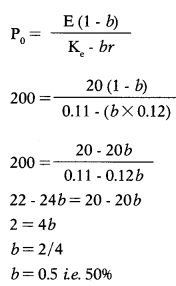Question 135.
Market price of Sara Ltd. is ₹ 1,000 per share. EPS is ₹ 20 per share. Cost of capital is 11 %. Rate of return on investment is 12%. What is dividend per share?
(A) 2
(B) 3
(C) 4
(D) 5
(A) 2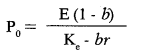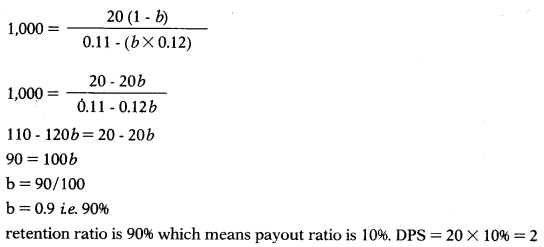Question 136.
Following information is available in respect of Sober Ltd.:
No. of shares outstanding : 1 lakh
Earnings per share : ₹ 4
Equity capitalization rate : 12%
Rate of return on investment : 15%
You are required to calculate Dividend payout ratio to keep share price at ₹ 40.
(A) 50%
(B) 40%
(C) 60%
(D) 20%
(D) 20%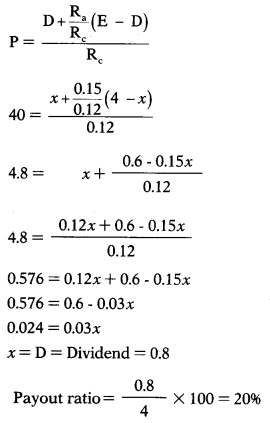Question 137.
A Chemical company belongs to a risk class for which P / E Ratio is 10. It currently has 50,000 equity shares selling at ₹ 200 each. The firm is contemplating the declaration of dividend of ₹ 16 per share at the current fiscal year which has just started. Given the assumption of Modigliani-Miller, what will be the price of share at the end of the year if dividend is declared?
(A) ₹ 205
(B) ₹ 208
(C) ₹ 204
(D) ₹ 225
(C) ₹ 204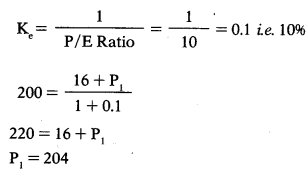Question 138.
Damodhar Ltd. has 10 lakh equity shares outstanding. Current market price of the shares is ₹ 150 each. The board of directors of the company has recommended dividend of ₹ 8 per share. Rate of capitalization is 12%. How many shares are to be issued as per MM Model at the end of accounting year on the assumption that the net income is ₹ 2 Crore and the investment budget is ₹ 4 Crore and dividend is declared as recommended by the directors.
(A) 1,19,047 shares
(B) 1,75,000 shares
(C) 1,57,000 shares
(D) 1,68,419 shares
(B) 1,75,000 shares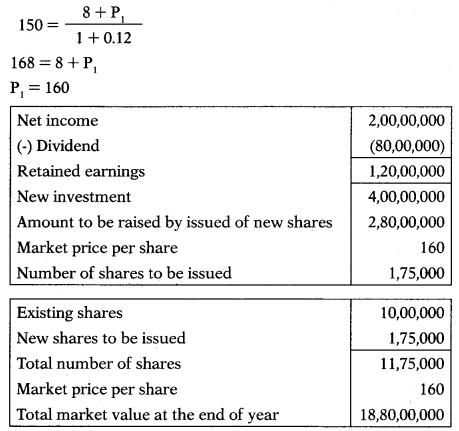Question 139.
Take the data above question and calculate the market value of the company after giving effect to proposal as stated above.
(A) ₹ 18,80,00,000
(B) ₹ 15,50,00,000
(C) ₹ 18,50,00,000
(D) ₹ 19,90,00,000
(A) ₹ 18,80,00,000Question 140.
An equity share of ₹ 100 is expected to earn an annual dividend of ₹10 and this share can be sold at price of ₹ 180 at the end of year. If the required rate of return is 12%, calculate the value of equity share.
(A) ₹ 196.46
(B) ₹ 169.64
(C) ₹ 149.66
(D) ₹ 170.05
(B) ₹ 169.64Question 141.
Net profit before tax of Acumen Ltd. is ₹ 17,50,000. The company has 1,00,000 equity shares of face value ₹ 10 each, fully paid-up. Current market price of the shares is ₹ 85 per share. Income-tax @ 30% applies to the company. Compute the P/E ratio for the company.
(A) 5.92
(B) 8.63
(C) 6.94
(D) 9.46
(C) 6.94Question 142.
Following details are available to you for Beauty Ltd.
Internal rate of return — 15%
Capitalization rate — 15%
Earnings per share — ₹ 12
Cash dividend per share — ₹ 5
Calculate the value of an equity share.
(A) ₹ 70
(B) ₹ 80
(C) ₹ 90
(D) ₹ 95
(B) ₹ 80Question 143.
Rama Ltd. had 1,00,000 equity shares of ₹ 10 each outstanding. Shares are currently being quoted at par in the market. In the wake of the removal of the dividend restraint, the company now intends to pay a dividend of ₹ 2 per share for the current financial year. It belongs to a risk class whose appropriate capitalization rate is 15%. Using MM Model and assuming no taxes, ascertain the price of the company’s shares as it is likely to prevail at the end of the year – (i) when dividend is declared; and (ii) when no dividend is declared.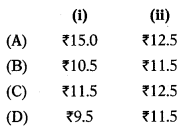(D)Question 144.
Rosa Ltd. has outstanding 1,20,000 shares selling at ₹ 20 per share. The company hopes to make a net income of ₹ 3,50,000 during the year. Company is thinking of paying a dividend of ₹ 2 per share at the end of current year. Capitalization rate has been estimated to be 15%. On the basis of MM model how many new shares the company must issue if the dividend is paid and company needs ?9,50,000 for an approved investment expenditure?
(A) 40,000 equity shares
(B) 50,000 equity shares
(C) 60,000 equity shares
(D) 70,000 equity shares
(A) 40,000 equity sharesQuestion 145.
Take the data of above, question and calculate total market value of the company.
(A) ₹ 35,40,000
(B) ₹ 32,90,000
(C) ₹ 33,60,000
(D) ₹ 34,30,000
(C) ₹ 33,60,000Question 146.
Abhishek Steel Ltd. has one lakh equity shares outstanding which are selling at ₹ 100 each. Its capitalization rate is 14%. The company is expecting ₹ 65 lakh income for the current year and is planning to pay dividend amounting to ₹ 4 lakh. The company wants to invest in a new project which will cost ₹ 75 lakh. It is assumed that the MM Model on dividend policy is applicable. Compute the number of shares to be issued for financing when: A. Dividend is not paid. B. Dividend amounting to ₹ 4 lakh is paid.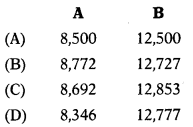(B)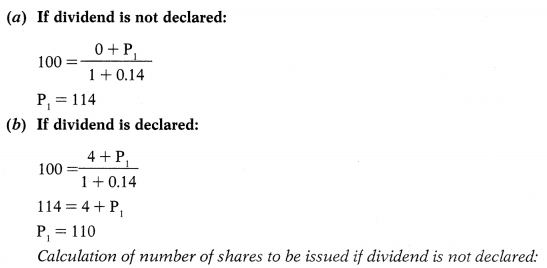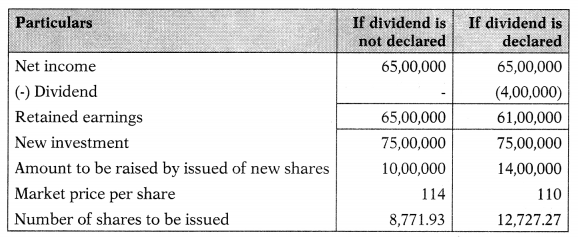Question 147.
Small Events Incorporation has recently paid dividend of ₹ 3.50 per share. The dividends are growing at 10% p.a. and the equity capitalization rate applicable to the company is 12%. Find out the implicit P/E Ratio if the EPS of the company is ₹ 7.
(A) 27.50
(B) 28.50
(C) 30.00
(D) 32.50
(A) 27.50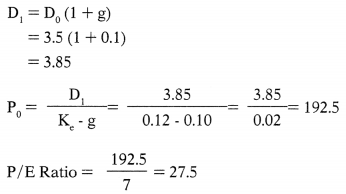Question 148.
Current price of share of X Ltd. is ₹ 60 and just paid dividend per share is ₹ 4. If the capitalization rate is 12%, what is the dividend growth rate?
(A) 3%
(B) 5%
(C) 4%
(D) 6%
(B) 5%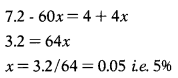Question 149.
Following information is available in respect of Hajela Ltd.:
No. of shares outstanding : 3 lakh
Net profit : 18 lakh
Equity capitalization rate : 16%
Rate of return on investment : 20%
You are required to calculate Dividend payout ratio to keep share price at ₹ 42.
(A) 42 %
(B) 46%
(C) 58%
(D) 52%